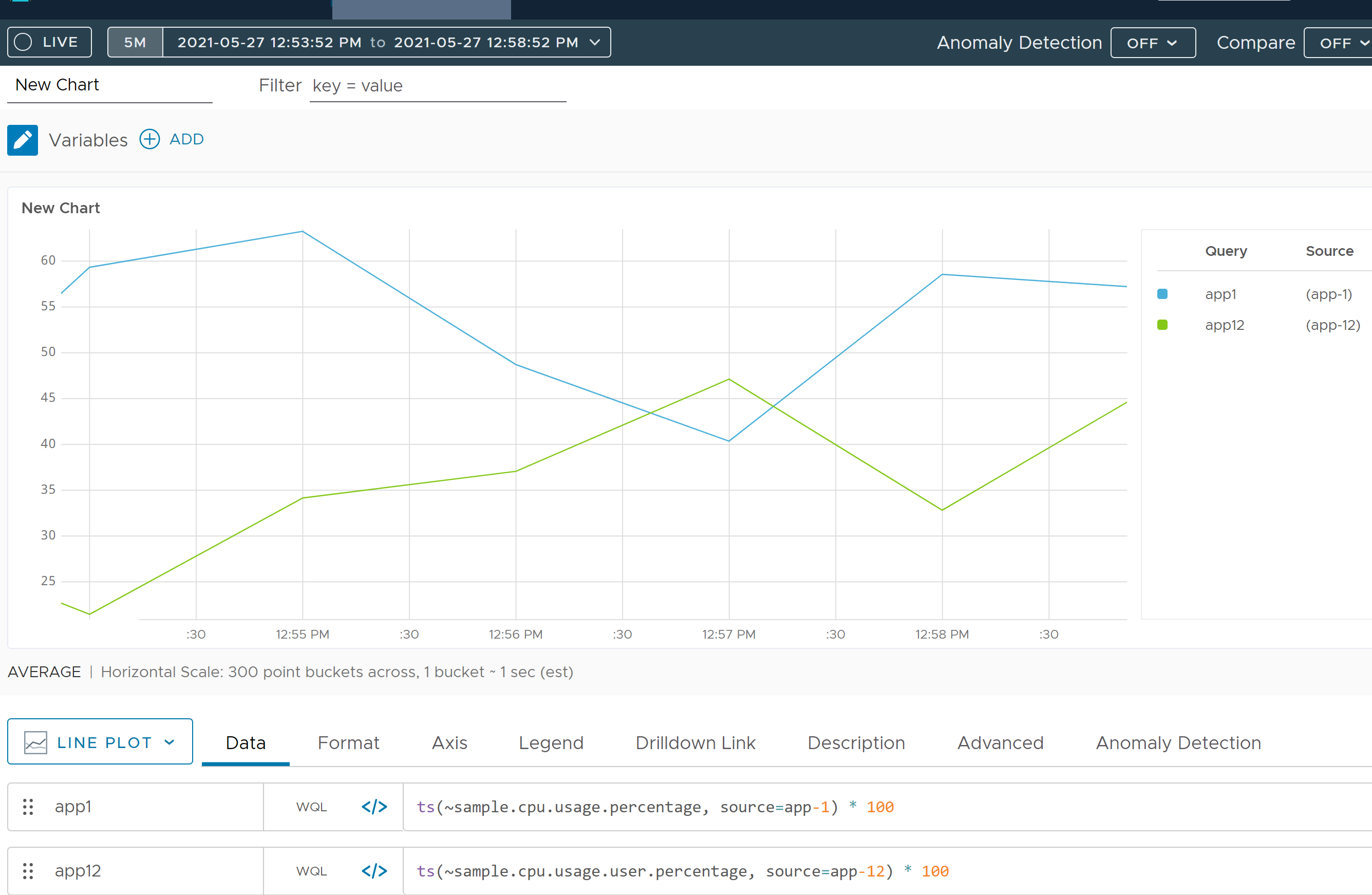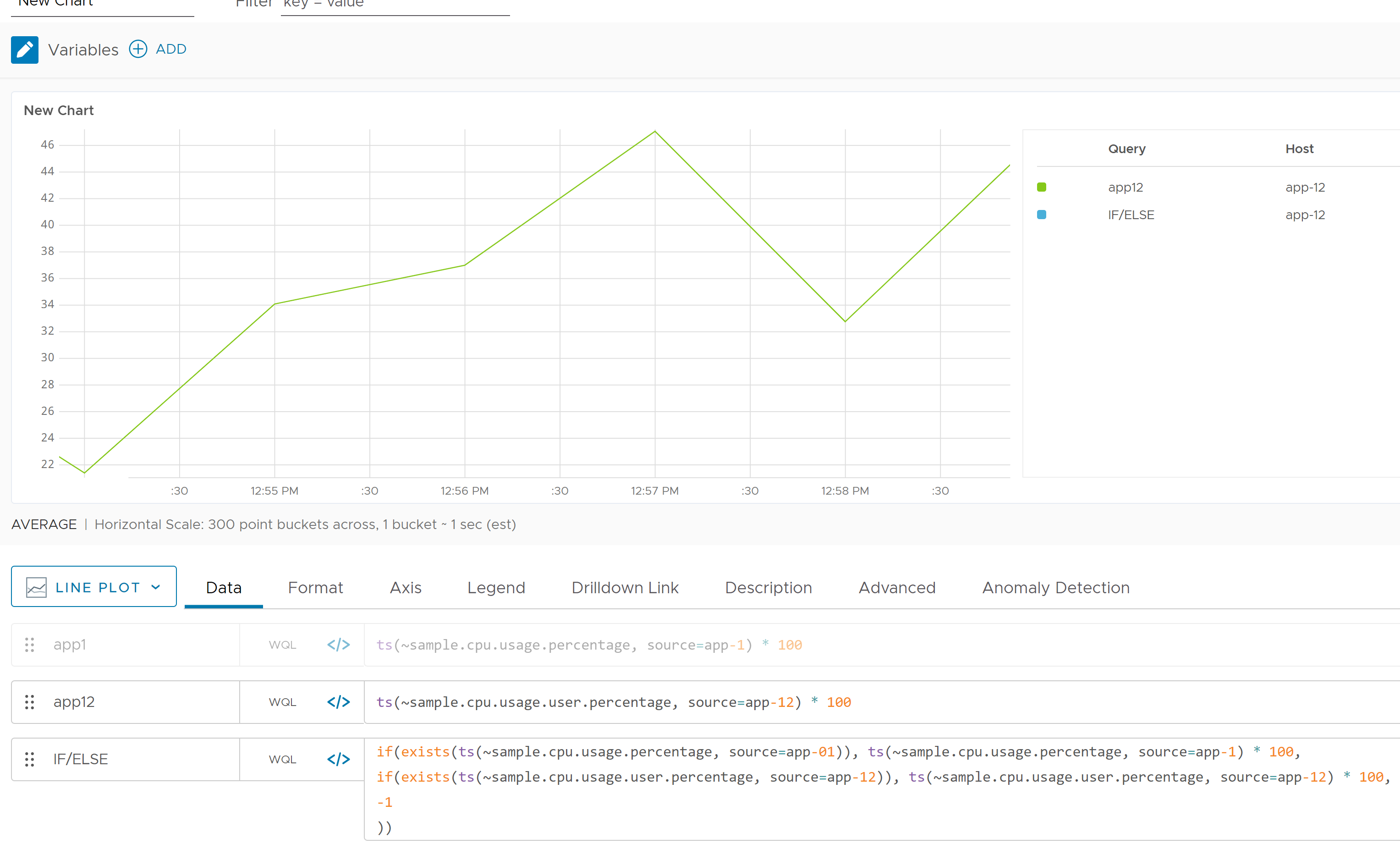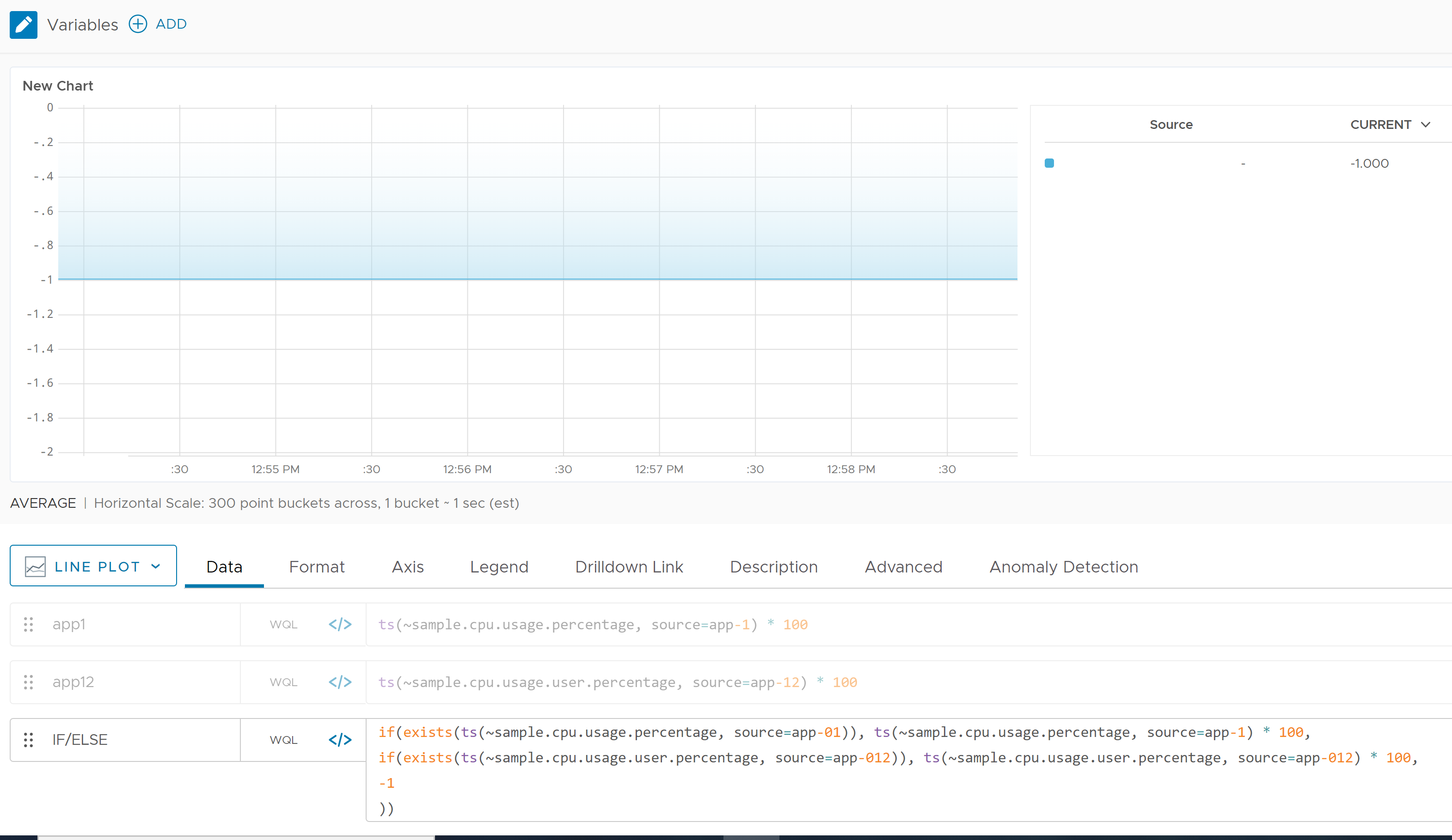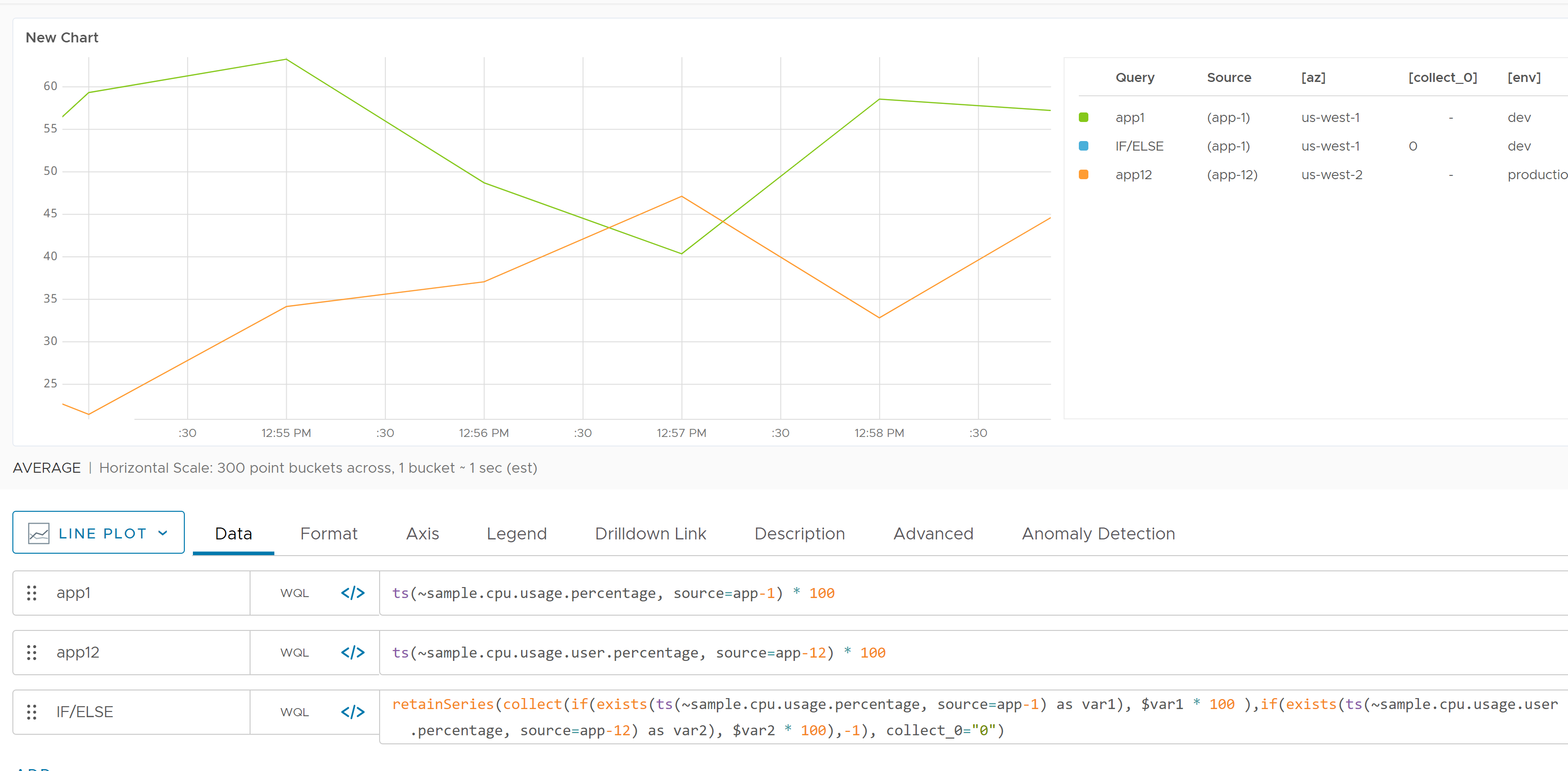Reference to the exists() function

## Summary

``````exists(<tsExpression>)
``````

Returns 1 if any time series described by the expression exists, and returns 0 otherwise. A time series exists if it has reported a data value in the last day (24 hours).

## Parameters

ParameterDescription
tsExpression Expression describing the time series to be tested for existence.

## Description

The `exists()` function returns 1 if any time series described by the expression exists, and returns 0 otherwise. A time series exists if it has at least one value that was reported within the last day (24 hours). The returned constant series is continuous.

You can use `exists()` to see if a metric is obsolete or has never reported.

`exists()` is useful if you’re creating a query that uses a metric that is planned but has not yet started reporting, for example, when you’re building a dashboard before any data is sent. Unless you wrap the query in `exists()`, all your charts will show errors for the missing metric. Using `exists` allows any syntax errors to be reported, which help you make corrections, but suppresses the errors for the missing metric.

## Examples

You can wrap `exists()` around an expression that returns some number of time series, like this:

``````exists(ts(inv_1_get_count, status="7**" and operation="*" and cname="\${environment}"))
``````

This query returns 1 if at least one time series has a value reported within the 1d (24 hours). Otherwise, the query returns 0 if the values of all of the time series are older than 1d (24 hours). Because this query returns either 1 or 0, it is useful as a conditional expression in an `if()` function.

For clarity, we’ll name this query `hasData` so we can easily refer to it in the example below.

This example tests `hasData` to see whether its underlying metrics are reporting data, and uses the result to choose between two other previously defined queries named `queryOfInterest` and `substituteQuery`:

``````if(\${hasData}, \${queryOfInterest}, \${substituteQuery})
``````

In this example:

• `hasData` evaluates to 1 (true) if its underlying metrics are reporting, so `if()` returns the results of `queryOfInterest`.
• `hasData` evaluates to 0 (false) if its underlying metrics are not reporting, so `if()` returns the results of `substituteQuery`.

## Using Exists() with Nested if/else Statements

When you create a query with exists(), you sometimes do not get the result you expected. The reason is that series matching occurs if a query uses exists() with nested if/else statements, and both expressions return data. This section explains how to get for results without series matching.

### Use Case

We’ll use the sample metric data `~sample.cpu.usage.percentage` and `~sample.cpu.usage.user.percentage` to demonstrate the nested if/else series matching in action.

The following screenshot uses the two sample metrics coming from two sources, app-1 and app-12. Both metrics values are multiplied by 100 for demonstration purposes.Suppose:

• You want to display the `~sample.cpu.usage.percentage` metric from app-1 (multiplied by 100), if the metric exists.
• Else if the metric does not exist, you want to display `~sample.cpu.usage.user.percentage` from app-12 (multiplied by 100), if that metric exists.
• If neither metric exists you want to display -1.

Here’s how that query looks (we’ve included line breaks for legibility):

``````if(exists
(ts(~sample.cpu.usage.percentage, source=app-1)),
ts(~sample.cpu.usage.percentage, source=app-1) * 100,
if(exists
(ts(~sample.cpu.usage.user.percentage, source=app-12)),
ts(~sample.cpu.usage.user.percentage, source=app-12) * 100,
-1))

``````

To test the query output, let’s use this query and make the first if statement false by using a source that doesn’t exist, app-01.

``````if(exists
(ts(~sample.cpu.usage.percentage, source=app-01)),
ts(~sample.cpu.usage.percentage, source=app-1) * 100,
if(exists
(ts(~sample.cpu.usage.user.percentage, source=app-12)),
ts(~sample.cpu.usage.user.percentage, source=app-12) * 100,
-1))
``````

Because app-01 doesn’t exist, we expect to see only the line for `~sample.cpu.usage.user.percentage`. The screenshot below verifies that’s what we see.If we use a source that doesn’t exist with both queries, we expect to see `-1`:

``````if(exists
(ts(~sample.cpu.usage.percentage, source=app-01)),
ts(~sample.cpu.usage.percentage, source=app-1) * 100,
if(exists
(ts(~sample.cpu.usage.user.percentage, source=app-012)),
ts(~sample.cpu.usage.user.percentage, source=app-012) * 100,
-1))
``````So the nested if/else statements work as expected when one or more of the metrics does not exist.

However, when both metrics exist in a nested if/else expression, then the results you see can be confusing because series matching occurs. In the following screenshot, the if/else query should show the value for app-1 (first condition) but shows the value for app-12 (second condition).### Workaround for Exists() Corner Cases

One way to workaround the `exists()` and nested if/else series matching problem, is to use a combination of the `retainSeries()` and `collect()` functions to ensure the displayed output follows a traditional nested if/else logical output.

With the same metrics we used above, we could write the following query (including line breaks for legibility):

``````retainSeries(collect(
if(exists
(ts(~sample.cpu.usage.percentage, source=app-1) as var1), \$var1 * 100 ),
if(exists
(ts(~sample.cpu.usage.user.percentage, source=app-12) as var2), \$var2 * 100),
-1),
collect_0="0")
``````

This expression ensures that the nested if/else works correctly for all queries.The `missing()` function lets you check whether a function returned data in a specified time window.